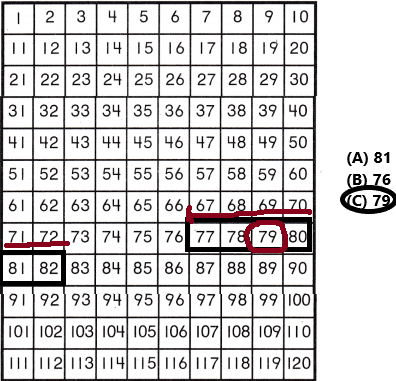# Texas Go Math Grade 1 Lesson 10.2 Answer Key Count Backward by Ones from 120

Refer to our Texas Go Math Grade 1 Answer Key Pdf to score good marks in the exams. Test yourself by practicing the problems from Texas Go Math Grade 1 Lesson 10.2 Answer Key Count Backward by Ones from 120.

## Texas Go Math Grade 1 Lesson 10.2 Answer Key Count Backward by Ones from 120

Explore

Count backward from 30. Write the numbers as you count backward.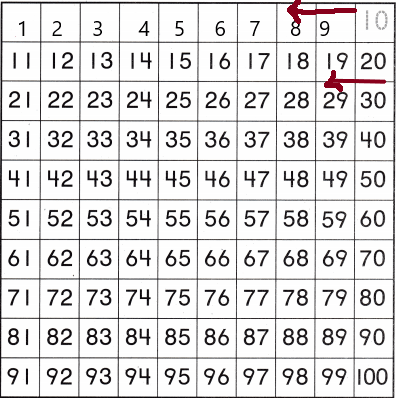Explanation:
We can count down or back by saying numbers in reverse order.

FOR THE TEACHER • Read the following problem. Miss Simmons is checking off the children as they leave the room. One row of numbers is incomplete. Use what you know about counting backward to write the numbers.
Counting Back – We can count down or back by saying numbers in reverse order while touching on each object once.
Here, for instance, we can reverse count the buttons by touching each button once.Counting back requires us to count backwards. Backward counting is counting by removing one, every time. Here, we reverse count while taking out the buttons from the jar, to find the number of the buttons.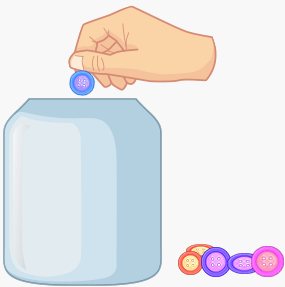Math Talk
Mathematical Processes

Explain how you know which numbers to write.
Answer: In backward counting, we need to write numbers in descending order.
For example, take numbers1, 2, 3, 4, 5, 6, 7, 8, 9, 10.
Here are these numbers, we need to write in backward counting. So we can write in descending order means big to small.
The backward counting is 10, 9, 8, 7, 6, 5, 4, 3, 2, 1.

Model and Draw

Count backwards. Write the numbers.
10 , 9 , ________, ________, ________
100, 99, ________, ________, ________
110, 109, ________, ________, ________By using counting track:
Backward counting means writing in a descending order which is big to small. We need to write in reverse order.

Share and Show

Use a Counting Chart. Count backward. Write the numbers.

Question 1.
114, ________, ________, ________, ________, ________, ________
By using the counting track write the numbers in backward counting.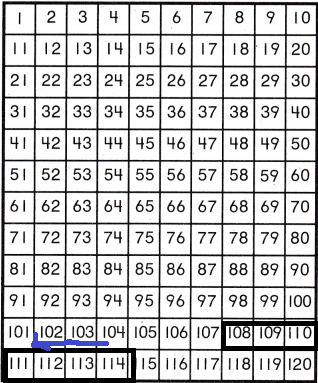114, 113, 112, 111, 110, 109, 108.
We need to write in a descending order that means big to small.

Question 2.
51, ________, ________, ________, ________, ________, ________
Answer: 51, 50, 49, 48, 47, 46, 45.Question 3.
94, ________, ________, ________, ________, ________, ________
Answer: 94, 93, 92, 91, 90, 89, 88.
Write in descending order.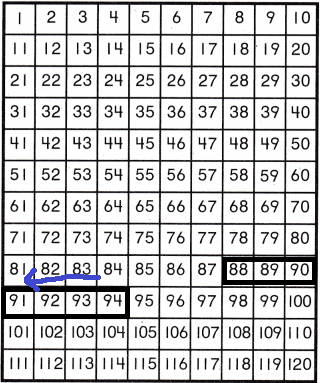Question 4.
78, ________, ________, ________, ________, ________, ________
Answer: 78, 77, 76, 75, 74, 73, 72.
In backward counting, the numbers always are in descending order that means the numbers are from big to small.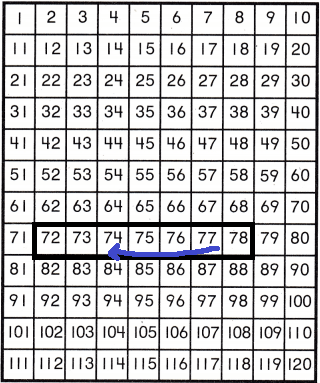Question 5.
35, ________, ________, ________, ________, ________, ________
Answer: 35, 34, 33, 32, 31, 30,29.
In backward counting, the numbers always are in descending order that means the numbers are from big to small.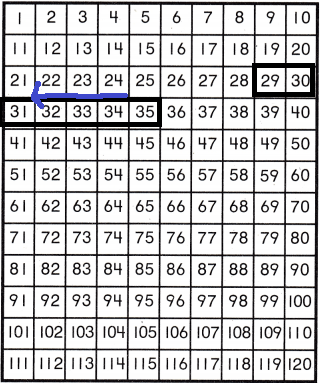Question 6.
109, ________, ________, ________, ________, ________, ________
Answer: 109, 108, 107, 106, 105, 104, 103.
In backward counting, the numbers always are in descending order that means the numbers are from big to small.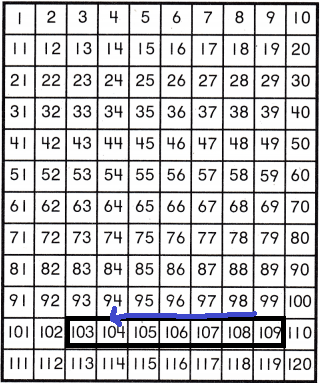Problem Solving

Use a Counting Chart. Count backward. Write the numbers.

Question 7.
19, ________, ________, ________, ________, ________, ________, ________, ________
Answer: 19, 18, 17, 16, 15, 14, 13, 12, 11.
In backward counting, the numbers always are in descending order that means the numbers are from big to small.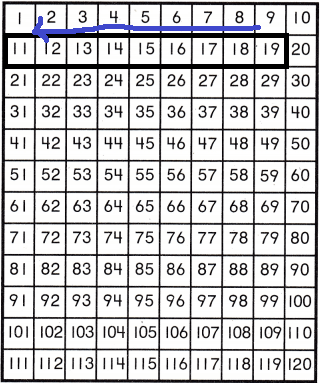Question 8.
86, ________, ________, ________, ________, ________, ________, ________, ________
Answer: 86, 85, 84, 83, 82, 81, 80, 79, 78.
In backward counting, the numbers always are in descending order that means the numbers are from big to small.Question 9.
107, ________, ________, ________, ________, ________, ________, ________, ________
Answer: 107, 106, 105, 104, 103, 102, 101, 100, 99.
In backward counting, the numbers always are in descending order that means the numbers are from big to small.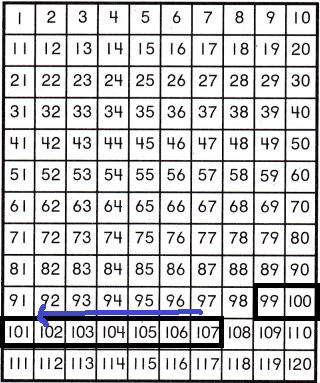Question 10.
43, ________, ________, ________, ________, ________, ________, ________, ________
Answer: 43, 42, 41, 40, 39, 38, 37,36, 35.
In backward counting, the numbers always are in descending order that means the numbers are from big to small.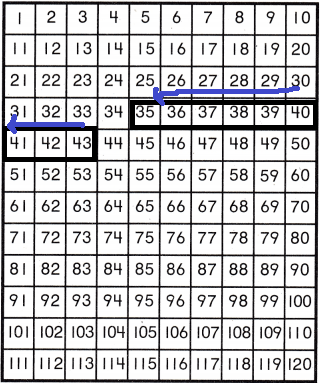Question 11.
68, ________, ________, ________, ________, ________, ________, ________, ________
Answer: 68, 67, 66, 65, 64, 63, 62, 61, 60.
In backward counting, the numbers always are in descending order that means the numbers are from big to small.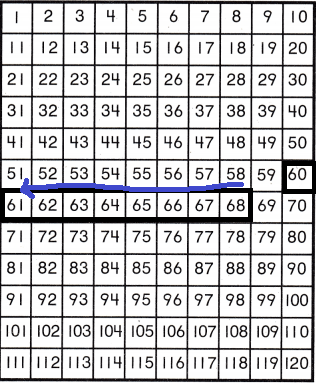Question 12.
22, ________, ________, ________, ________, ________, ________, ________, ________
Answer: 22, 21, 20, 19, 18, 17, 16, 15,14.
In backward counting, the numbers always are in descending order that means the numbers are from big to small.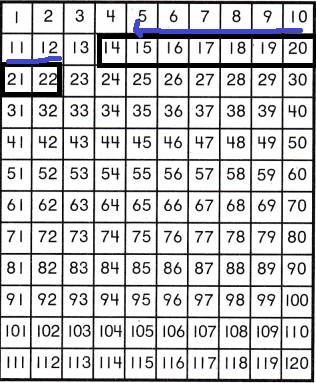Question 13.
H.O.T. Use a Counting Chart to write the numbers counting backward.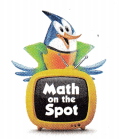120, ________, ________, ________, ________, ________
Answer: 120, 119, 118, 117, 116, 115.
In backward counting, the numbers always are in descending order that means the numbers are from big to small.Question 14.
H.O.T. Multi-Step There is an unknown number in the sequence counting backwards. The number is after 48. The number is before 46. What is the unknown number?
The unknown number in backward counting is after 48 and before 46. This representation will be in the below-counting track.Question 15.
Analyze Shawn is counting backward from 101. He is stuck at 100. Which numbers come next?
101, 100, ________, ________, ________, ________
(A) 95, 90, 85, 80
(B) 90, 80, 70, 60
(C) 99, 98, 97, 96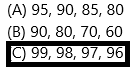Explanation:
In backward counting, the numbers always are in descending order that means the numbers are from big to small. Here he is counting from backward 101, 100, 99, 98, 97, 96…

Question 16.
Jackie has 25 toy cars. Each car has a number on it from 1 to 25. Jackie wants to put the cars in order from the greatest number to the least. She finds the first three cars. Which car number comes next?(A) 20
(B) 22
(C) 15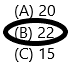Explanation:
He wants to put the car from greatest to least number. He selected the cars having numbers 15, 20, 22. The greatest number is 22 here so he selects the greatest number on a car because he is counting from backwards. The backward counting is always in descending order.

Question 17.
Dan left a number off the chart. Write the number that is not shown.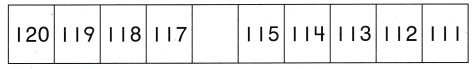Explanation:
In backward counting, the numbers always are in descending order that means the numbers are from big to small.

Question 18.
Texas Test Prep Count backward. What number is not shown?
120, 119, _____ , 117, 116
(A) 118
(B) 117
(C) 120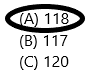Explanation:
In backward counting, the numbers always are in descending order that means the numbers are from big to small.
So the numbers are 120, 119, 118, 117, 116.

TAKE HOME ACTIVITY • Place 10 objects in a row. Hove your child point to each object as he or she counts backward from 10.Take any objects, here I take stars and make them count from backwards which is from greatest number to lowest number.

### Texas Go Math Grade 1 Lesson 10.2 Homework and Practice Answer Key

Use a Counting Chart. Count backward. Write the numbers.Question 1.
41, _______, _______, _______
Explanation:
In backward counting, the numbers always are in descending order that means the numbers are from big to small.Question 2.
83, _______, _______, _______
Explanation:
In backward counting, the numbers always are in descending order that means the numbers are from big to small.Question 3.
104, _______, _______, _______, _______, _______
Answer: 104, 103, 102, 101, 100, 99.
Explanation:
In backward counting, the numbers always are in descending order that means the numbers are from big to small.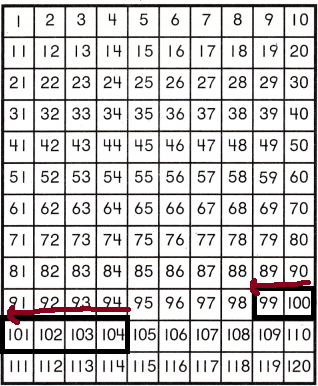Question 4.
30, _______, _______, _______, _______, _______, _______
Answer: 30, 29, 28, 27, 26, 25, 24.
Explanation:
In backward counting, the numbers always are in descending order that means the numbers are from big to small.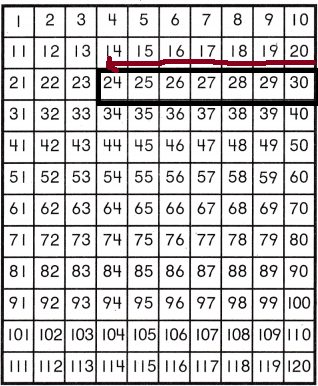Problem Solving

Use a Counting Chart.

Question 5.
Jed has a book with missing pages. Count backward. Fill in the numbers of the missing pages.
24, 23, _______, _______, 20, _______, _______
Answer: 24, 23, 22, 21, 20, 19, 18.
Explanation:
The Jed has a book having with some missed pages. Here we need to count backwards. The missing pages will be shown in the counting track diagram.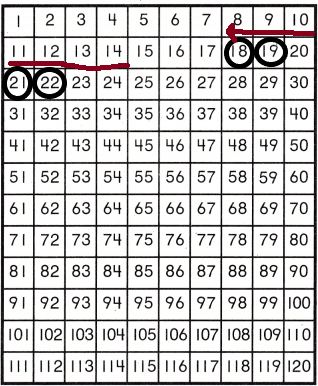Lesson Check

Question 6.
Andy is learning to count backward. His teacher left some numbers off the chart. Which numbers are not shown?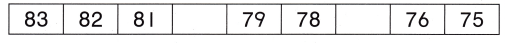(A) 79 and 82
(B) 70 and 75
(C) 80 and 77Explanation:
Counting backwards- some numbers are missed here that are 80 and 77.

Question 7.
Cal and Kim are counting backward from 43. They don’t agree over which numbers come next. Which numbers are correct?
43, 42, ________, ________, ________, _________
(A) 41, 40, 39, 38
(B) 43, 44, 45, 46
(C) 40, 30, 20, 10Explanation:
Cal and Kim, both are counting backwards from the number 43. They need to count in a descending order that means big number to small number.

Question 8.
Multi-Step Jack counted the stamps in his collection. The number of stamps is more than 61. The number is less than 63. How many stamps does he have?(A) 67
(B) 62
(C) 60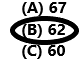Explanation:
In the question asked was the number which is more than 61 and less than 63. Definitely, the number is 62. The representation is shown in the counting tracks.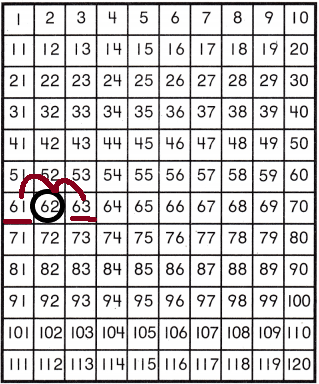Question 9.
Count backward. What number is not shown?
82, 81, 80, _________, 78, 77
(A) 81
(B) 76
(C) 79